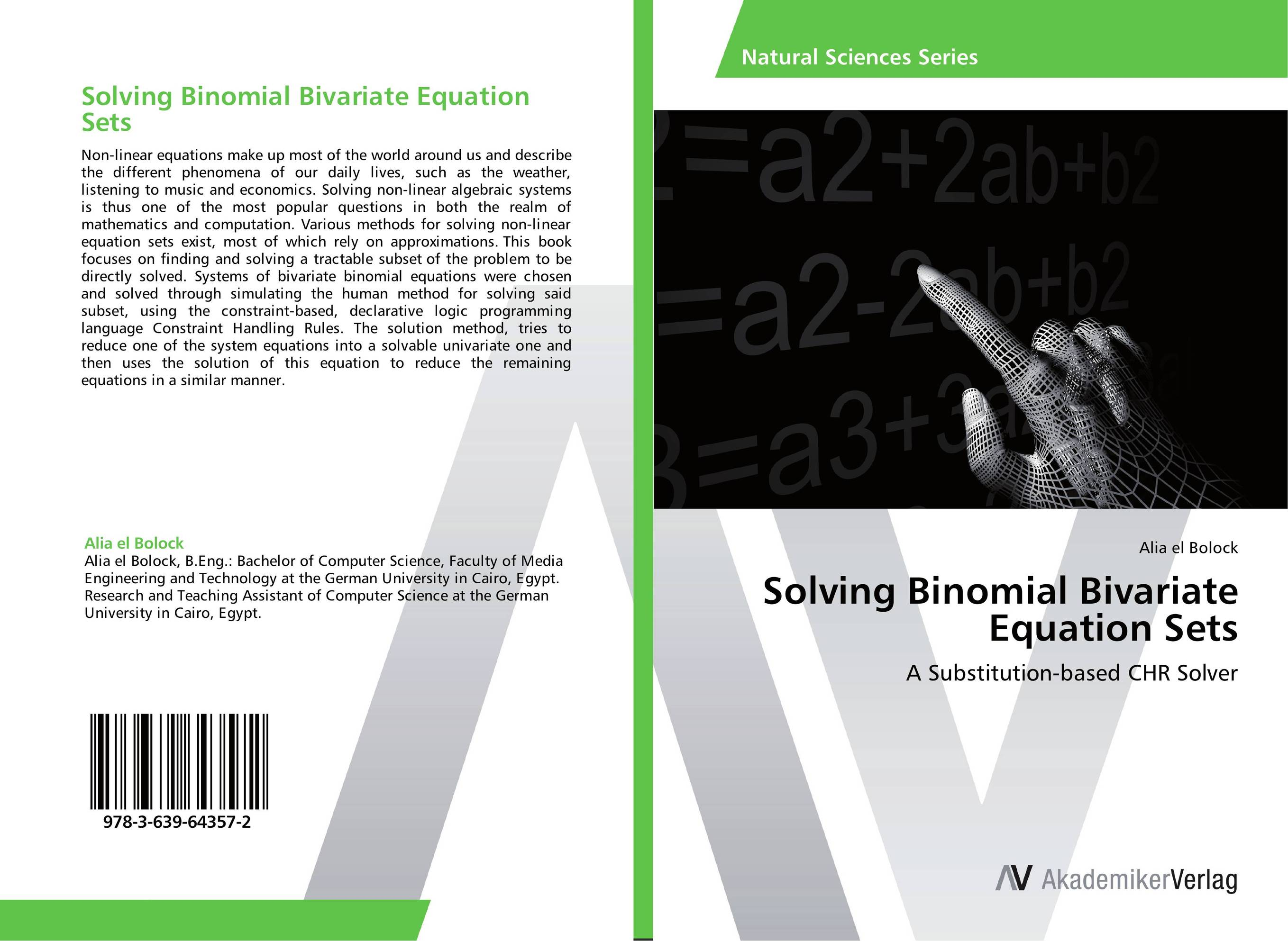# Solving Binomial Bivariate Equation Sets

###### Аннотация:
Non-linear equations make up most of the world around us and describe the different phenomena of our daily lives, such as the weather, listening to music and economics. Solving non-linear algebraic systems is thus one of the most popular questions in both the realm of mathematics and computation. Various methods for solving non-linear equation sets exist, most of which rely on approximations. This book focuses on finding and solving a tractable subset of the problem to be directly solved. Systems of bivariate binomial equations were chosen and solved through simulating the human method for solving said subset, using the constraint-based, declarative logic programming language Constraint Handling Rules. The solution method, tries to reduce one of the system equations into a solvable univariate one and then uses the solution of this equation to reduce the remaining equations in a similar manner.
###### Издания произведения:9783639643572

### Рекомендуем

#### Комментарии

Пока нет ни одного комментария
Вы должны войти для того что бы оставлять комментарии